Forces

In point O acts three orthogonal forces: F1 = 20 N, F2 = 7 N and F3 = 19 N. Determine the resultant of F and the angles between F and forces F1, F2 and F3.

Result

F =  28.5 N
α =  45.4 °
β =  75.8 °
γ =  48.1 °

Solution:Leave us a comment of example and its solution (i.e. if it is still somewhat unclear...):Be the first to comment!To solve this verbal math problem are needed these knowledge from mathematics:

For Basic calculations in analytic geometry is helpful line slope calculator. From coordinates of two points in the plane it calculate slope, normal and parametric line equation(s), slope, directional angle, direction vector, the length of segment, intersections the coordinate axes etc. Do you want to convert length units? Pythagorean theorem is the base for the right triangle calculator. Most natural application of trigonometry and trigonometric functions is a calculation of the triangles. Common and less common calculations of different types of triangles offers our triangle calculator. Word trigonometry comes from Greek and literally means triangle calculation.

Next similar examples:

1. Two peopleTwo straight lines cross at right angles. Two people start simultaneously at the point of intersection. John walking at the rate of 4 kph in one road, Jenelyn walking at the rate of 8 kph in the other road. How long will it take for them to be 20√5 km apar
2. Slope formFind the equation of a line given the point X(8, 1) and slope -2.8. Arrange your answer in the form y = ax + b, where a, b are the constants.
3. Right triangle from axesA line segment has its ends on the coordinate axes and forms with them a triangle of area equal to 36 sq. Units . The segment passes through the point ( 5,2). What is the slope of the line segment. ?
4. Three points 2The three points A(3, 8), B(6, 2) and C(10, 2). The point D is such that the line DA is perpendicular to AB and DC is parallel to AB. Calculate the coordinates of D.
5. Center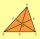In the triangle ABC is point D[1,-2,6], which is the center of the |BC| and point G[8,1,-3], which is the center of gravity of the triangle. Find the coordinates of the vertex A[x,y,z].
6. LineLine p passing through A[-10, 6] and has direction vector v=(3, 2). Is point B[7, 30] on the line p?
7. Points collinear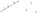Show that the point A(-1,3), B(3,2), C(11,0) are col-linear.
8. CuboidsTwo separate cuboids with different orientation in space. Determine the angle between them, knowing the direction cosine matrix for each separate cuboid. u1=(0.62955056, 0.094432584, 0.77119944) u2=(0.14484653, 0.9208101, 0.36211633)
9. LineStraight line passing through points A [-3; 22] and B [33; -2]. Determine the total number of points of the line which both coordinates are positive integers.
10. TriangleTriangle KLM is given by plane coordinates of vertices: K[-12, -11] L[-15, -18] M[-13, -12]. Calculate its area and itsinterior angles.
11. Cone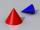If the segment of the line y = -3x +4 that lies in quadrant I is rotated about the y-axis, a cone is formed. What is the volume of the cone?
12. Find the 5Find the equation with center at (1,20) which touches the line 8x+5y-19=0
13. Slope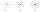Calculate the slope of a line that intersects points (-84,41) and (-76,-32).
14. Angle between vectorsFind the angle between the given vectors to the nearest tenth of a degree. u = (-22, 11) and v = (16, 20)
15. Perpendicular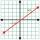What is the slope of the perpendicular bisector of line segment AB if A[-4,-5] and B[1,-1]?
16. Right angled triangle 2LMN is a right angled triangle with vertices at L(1,3), M(3,5) and N(6,n). Given angle LMN is 90° find n
17. Angle between lines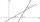Calculate the angle between these two lines: ? ?